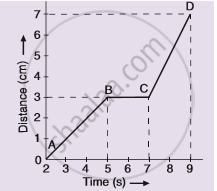# The Graph Given Alongside Shows the Positions of a Body at Different Times. Calculate the Speed of the Body as It Moves from : (I) a to B, (Ii) B to C, and (Iii) C to D - Science

Graph

The graph given alongside shows the positions of a body at different times. Calculate the speed of the body as it moves from :
(1) A to B,
(2) B to C, and
(3) C to D.#### Solution

(i) The distance covered from A to B,
= 3 – 0
= 3 cm
Time taken to cover the distance from A to B
= 5 – 2
= 3 s
Hence speed,
= "Distance"/"Time"
= 3/3 cm/s
= 1 cm/s
(ii) The speed of the body as it moves from B to C is zero because the distance travelled is zero.
(iii) The distance covered from C to D,
= 7 – 3
= 4 cm
Time taken to cover the distance from C to D,
= 9 – 7
= 2 s
Hence speed,
= "Distance"/"Time"
= 4/2  cm/s
= 2 cm/s

Concept: Graphical Representation of Linear Motion - Distance - Time Graphs
Is there an error in this question or solution?

#### APPEARS IN

Lakhmir Singh Science Class 9 Physics
Chapter 1 Motion
Hots Questions | Q 53 | Page 43